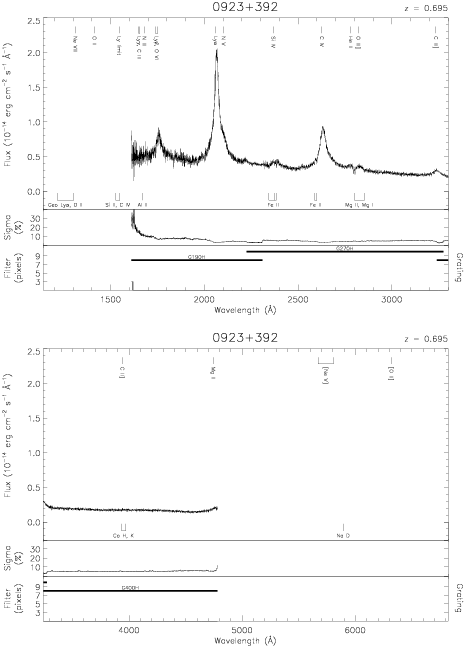Download dataset
TELESCOP= 'HST     '
INSTRUME= 'FOS     '
RADECSYS= 'FK5     '
EQUINOX =               2000.0
DATE-OBS= '1993-01-24T11:27:47'
RA_PNT  =       141.7627083333
DEC_PNT =        39.0391388889
PA_PNT  =       315.4487000000
OBJECT  = '0923+392'
ROOTNM01= 'Y1170102T'
GRNDMD01= 'SPECTROSCOPY'
DETECT01= 'AMBER   '
APERID01= 'A-1     '
FGWAID01= 'H19     '
ROOTNM02= 'Y1170103T'
GRNDMD02= 'SPECTROSCOPY'
DETECT02= 'AMBER   '
APERID02= 'A-1     '
FGWAID02= 'H27     '
ROOTNM03= 'Y1170104T'
GRNDMD03= 'SPECTROSCOPY'
DETECT03= 'AMBER   '
APERID03= 'A-1     '
FGWAID03= 'H40     '